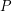# Drawings

## Universal point sets for planar graphs ★★★

Author(s): Mohar

We say that a setis-universal if everyvertex planar graph can be drawn in the plane so that each vertex maps to a distinct point in, and all edges are (non-intersecting) straight line segments.

Question   Does there exist an-universal set of size?

Keywords: geometric graph; planar graph; universal set

## Linear Hypergraphs with Dimension 3 ★★

Author(s): de Fraysseix; Ossona de Mendez; Rosenstiehl

Conjecture   Any linear hypergraph with incidence poset of dimension at most 3 is the intersection hypergraph of a family of triangles and segments in the plane.

Keywords: Hypergraphs